## Use Variant Control Variables in Variant Blocks

This topic explains how to use different types of variant control variables in variant blocks.

Consider the `slexVariantSubsystems` model.The `Controller` subsystem block dialog specifies two potential variants `Linear Controller` and ```Nonlinear Controller```. `Linear Controller` and ```Nonlinear Controller``` blocks are associated with variant condition expressions `V == 1` and `V == 2`. Here, `V` is the variant control variable that determines the active choice. You can change `V` to any of these types based on your requirement.

### Simulink.VariantControl Variables for Coherent Switching of Choices in Variant Blocks

You can associate a variant control variable of type `Simulink.VariantControl` with a variant activation time. `Simulink.VariantControl` variant control variables help you switch variant elements such as blocks and parameters coherently.

Consider a group of blocks that represents a design choice, with each block having the same variant control variable of type `Simulink.VariantControl`. If you set the variant activation time of these blocks to `inherit from Simulink.VariantControl`, the blocks inherit the activation time from the variant control variable. As a result, all the blocks have the same activation time and are activated simultaneously to generate rational simulation results and valid code.

You can define `Simulink.VariantControl` type of control variables in storage locations as listed in Storage Locations for Variant Control Variables (Operands) in Variant Blocks.

Open the `slexVariantSubsystems` model.

`open_system('slexVariantSubsystems');`
```VSS_LINEAR_CONTROLLER = VariantExpression with properties: Condition: 'VSS_MODE==1' ```
```VSS_NONLINEAR_CONTROLLER = VariantExpression with properties: Condition: 'VSS_MODE==2' ```
```VSS_MODE = 2 ```

In the block parameters dialog box of the `Controller` block:

1. Specify the variant controls, `V == 1` and `V == 2`.

```set_param('slexVariantSubsystems/Controller/Linear Controller', 'VariantControl', 'V==1') set_param('slexVariantSubsystems/Controller/Nonlinear Controller', 'VariantControl', 'V==2')```

2. Set Variant activation time to `inherit from Simulink.VariantControl` to inherit the activation time from `V`.

`set_param('slexVariantSubsystems/Controller', 'VariantActivationTime', 'inherit from Simulink.VariantControl')`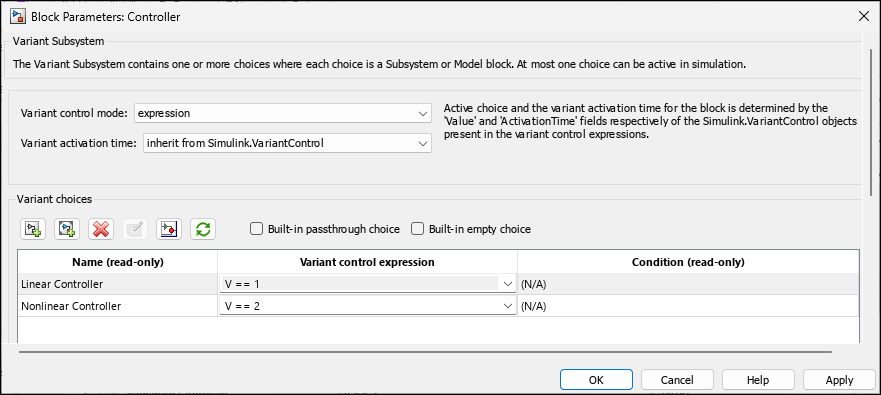In the base workspace, define a `Simulink.VariantControl` object, `V`. Set its value to `1`, variant activation time to `update diagram`, and then simulate the model.

`V = Simulink.VariantControl('Value', 1, 'ActivationTime', 'update diagram')`
```V = VariantControl with properties: Value: 1 ActivationTime: 'update diagram' ```

During simulation, the `Controller` block inherits the `update diagram` activation time from `V` and the `Linear Controller` block becomes active.

Double-click the `Controller` block to see the active choice.

`sim ('slexVariantSubsystems');`

If you change the value of `V` to `2`, the `Nonlinear Controller` block becomes active during simulation. You can change the value of `V` using this command or from `Simulink.VariantControl` dialog box.

```V.Value = 2; sim ('slexVariantSubsystems');```

If you change the variant activation time of `V` to `update diagram analyze all choices`, the `Controller` block inherits the `update diagram analyze all choices `activation time from `V`. You can observe the inherited activation time using `CompiledVariantActivationTime`.

```V.ActivationTime = 'update diagram analyze all choices'; sim ('slexVariantSubsystems'); get_param('slexVariantSubsystems/Controller', 'CompiledVariantActivationTime')```
```ans = 'update diagram analyze all choices' ```

### Scalar Variant Control Variables for Rapid Prototyping in Variant Blocks

Scalar MATLAB® variant control variables allow you to rapidly prototype variant choices when you are still building your model. Scalar MATLAB variant control variables help you focus more on building your variant choices than on developing the expressions that activate those choices.

You can define scalar control variables in storage locations as listed in Storage Locations for Variant Control Variables (Operands) in Variant Blocks.

Open the `slexVariantSubsystems` model.

`open_system('slexVariantSubsystems');`
```VSS_LINEAR_CONTROLLER = VariantExpression with properties: Condition: 'VSS_MODE==1' ```
```VSS_NONLINEAR_CONTROLLER = VariantExpression with properties: Condition: 'VSS_MODE==2' ```
```VSS_MODE = 2 ```

In the block parameters dialog box of the `Controller` block, specify variant controls in their simplest form as scalar variables, `V == 1` and `V == 2`.

```set_param('slexVariantSubsystems/Controller/Linear Controller', 'VariantControl', 'V==1') set_param('slexVariantSubsystems/Controller/Nonlinear Controller', 'VariantControl', 'V==2')```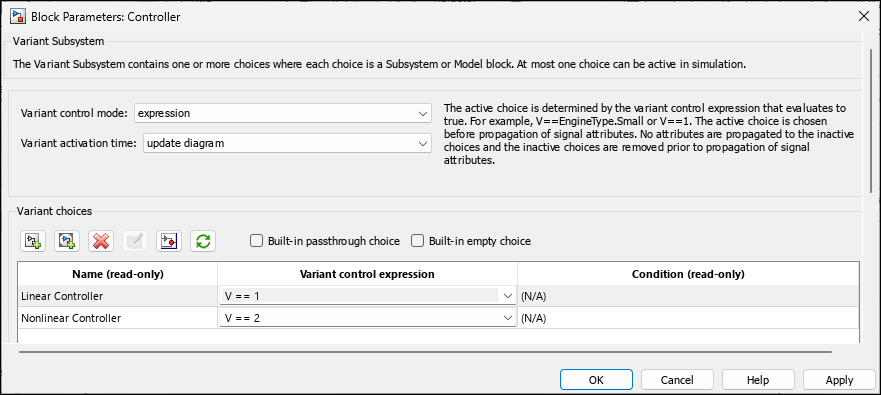In the base workspace, define a variant control variable, `V`, set its value to `1`, and then simulate the model.

`V = 1;`

During simulation, the `Linear Controller` block becomes active. Double-click the `Controller` block to see the active choice.

`sim ('slexVariantSubsystems');`

Similarly, if you change the value of `V` to `2`, the `Nonlinear Controller` block becomes active during simulation.

```V = 2; sim ('slexVariantSubsystems');```

### `Simulink.Parameter` Type of Variant Control Variables for Code Generation in Variant Blocks

If you intend to generate code for a model containing variant blocks, specify variant control variables as `Simulink.Parameter` objects. `Simulink.Parameter` objects allow you to specify other attributes, such as data type and storage class, and control the appearance and placement of variant control variables in generated code.

• You can define a variant control variable of type `Simulink.Parameter` only in the base workspace or in a data dictionary. Defining `Simulink.Parameter` type of variant control variables in the mask or model workspace is not supported. For more information on storage locations for variant control variables, see Storage Locations for Variant Control Variables (Operands) in Variant Blocks.

• `Simulink.Parameter` objects within structures and that have data types other than `Simulink.Bus` objects are not supported.

Open the `slexVariantSubsystems` model.

`open_system('slexVariantSubsystems');`
```VSS_LINEAR_CONTROLLER = VariantExpression with properties: Condition: 'VSS_MODE==1' ```
```VSS_NONLINEAR_CONTROLLER = VariantExpression with properties: Condition: 'VSS_MODE==2' ```
```VSS_MODE = 2 ```

In the MATLAB Editor, define a `Simulink.Parameter` object, `V`.

```V = Simulink.Parameter; V.Value = 1; V.DataType = 'int32'; V.CoderInfo.StorageClass = 'Custom'; V.CoderInfo.CustomStorageClass = 'ImportedDefine'; V.CoderInfo.CustomAttributes.HeaderFile ='rtwdemo_importedmacros.h';```

Note:

Variant control variables defined as `Simulink.Parameter` objects can have any of the storage classes listed in Storage Classes for Different Variant Activation Times.

You can also convert a scalar variant control variable into a `Simulink.Parameter` object. For more information, see Convert Variant Control Variables into Simulink.Parameter Objects.

Specify the `Simulink.Parameter` object as the variant control variable in the block parameters dialog box of the Controller block. Also, change the Variant activation time to `code compile`.

```set_param('slexVariantSubsystems/Controller/Linear Controller', 'VariantControl', 'V==1') set_param('slexVariantSubsystems/Controller/Nonlinear Controller', 'VariantControl', 'V==2') set_param('slexVariantSubsystems/Controller', 'VariantActivationTime', 'code compile')```

Ensure that the `Linear Controller` and `NonLinear Controller` blocks are atomic.

```set_param('slexVariantSubsystems/Controller/Linear Controller', 'TreatAsAtomicUnit', 'on'); set_param('slexVariantSubsystems/Controller/Nonlinear Controller', 'TreatAsAtomicUnit', 'on');```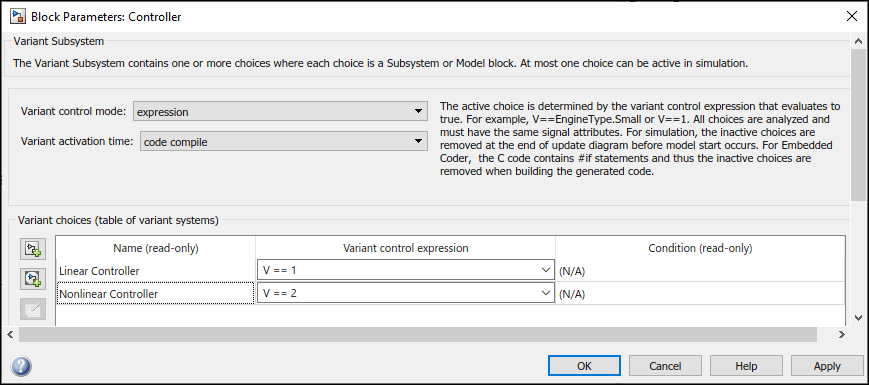During simulation, the `Linear Controller` block becomes active. Double-click the `Controller` block to see the active choice.

`sim ('slexVariantSubsystems');`

Similarly, if you change the value of `V` to `2`, the `Nonlinear Controller` block becomes active during simulation.

```V .Value = 2; sim ('slexVariantSubsystems');```

Generate code from the model. For information on how to generate code, see Generate Code Using Embedded Coder (Embedded Coder).

The generated code contains both Linear and Nonlinear choices in preprocessor conditionals `#if` and `#elif` because of the `code compile` activation time. The variant control variable `V` is defined using a macro — `#define` directive — in the header file `demos_macro.h`. You can control the appearance and placement of `V` in the generated code and prevent optimizations from eliminating storage for `V` using the storage class property. For more information, see Storage Classes for Different Variant Activation Times.

```% demos_macro.h % /* Exported data define */ % % /* Definition for custom storage class: Define */ % #define V 1 /* Referenced by: % * '<S1>/Linear Controller' % * '<S1>/Nonlinear Controller' % */ % #endif /* RTW_HEADER_demo_macros_h_ */```

### Enumerated Types To Improve Code Readability of Variant Control Variables of Variant Blocks

Use enumerated types to give meaningful names to integers used as variant control values. For more information on enumerated type data, see Use Enumerated Data in Simulink Models.

In the MATLAB® Editor, define the classes that map enumerated values to meaningful names.

Open the `slexVariantSubsystems` model.

`open_system('slexVariantSubsystems');`
```VSS_LINEAR_CONTROLLER = VariantExpression with properties: Condition: 'VSS_MODE==1' ```
```VSS_NONLINEAR_CONTROLLER = VariantExpression with properties: Condition: 'VSS_MODE==2' ```
```VSS_MODE = 2 ```

In the base workspace, define the classes that map enumerated values to meaningful names.

Here, `EngType` is an integer-based enumeration class that is derived from the built-in data type, `int32`. The class has two enumeration values, `Linear` and `NonLinear`. These enumerated values have underlying integer values `0` and `1`.

`type EngType`
```classdef EngType < Simulink.IntEnumType enumeration Small (1) Big (2) end methods (Static) function retVal = addClassNameToEnumNames() % ADDCLASSNAMETOENUMNAMES Specifies whether to add the class name % as a prefix to enumeration member names in generated code. % Return true or false. % If you do not define this method, no prefix is added. retVal = true; end end end ```

Specify the enumeration names as variant control variables in the block parameters dialog box of the `Controller` block. Also, change the Variant activation time to `code compile`.

```set_param('slexVariantSubsystems/Controller/Linear Controller', 'VariantControl', 'V==EngType.Small') set_param('slexVariantSubsystems/Controller/Nonlinear Controller', 'VariantControl', 'V==EngType.Big') set_param('slexVariantSubsystems/Controller', 'VariantActivationTime', 'code compile')```

Ensure that the `Linear Controller` and `NonLinear Controller` blocks are atomic.

```set_param('slexVariantSubsystems/Controller/Linear Controller', 'TreatAsAtomicUnit', 'on'); set_param('slexVariantSubsystems/Controller/Nonlinear Controller', 'TreatAsAtomicUnit', 'on');```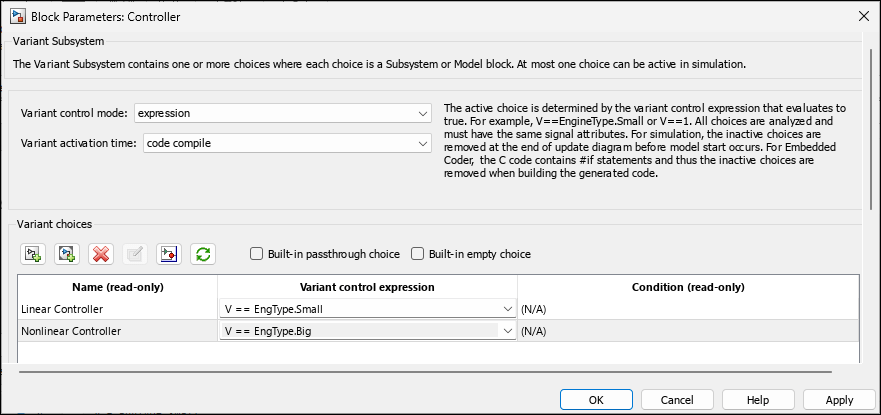Define the variant control variable, `V` in the base workspace, specify its value as `EngType.Small` and then simulate the model.

`V = EngType.Small;`

During simulation, the `Linear Controller` block becomes active. Double-click the `Controller` block to see the active choice.

`sim ('slexVariantSubsystems');`

The code that you generate using enumerated types contains the names of the values rather than integers. Here, values `1` and `2` are represented as `EngType_Small` and `EngType_Big`.

```% slexVariantSubsystems_types.h % #ifndef V % #define V EngType_Small % #endif % % #ifndef DEFINED_TYPEDEF_FOR_EngType_ % #define DEFINED_TYPEDEF_FOR_EngType_ % % typedef int32_T EngType; % % /* enum EngType */ % #define EngType_Small (1) /* Default value */ % #define EngType_Big (2) % #endif % slexVariantSubsystems.c % void slexVariantSubsystems_step(void) % { % #if V == EngType_Small % logic of Linear Controller % #elif V == EngType_Big % logic of Nonlinear Controller % #endif % } ```

Note that for variant blocks with startup activation time, only enumerations that are defined using these techniques are supported:

• Using the function `Simulink.defineIntEnumType`

• By subclassing built-in integer data types `int8`, `int16`, `int32`, `uint8`, or `uint16`, or by subclassing `Simulink.IntEnumType`

These enumerations are also supported when permanently stored in a Simulink® data dictionary. See Enumerations in Data Dictionary.

### Simulink.Variant Objects for Variant Condition Reuse of Variant Blocks

After identifying the variant values that your model requires, you can construct complex variant conditions to control the activation of your variant blocks by defining variant conditions as `Simulink.Variant` objects. `Simulink.Variant` objects enable you to reuse common variant conditions across models and help you encapsulate complex variant condition expressions.

You can specify the whole of a variant condition expression or only the variant control variable inside the `Condition` property of the `Simulink.Variant` object.

Note:

• You can define a variant control variable of type `Simulink.Variant` only in the base workspace or in a data dictionary. Defining variant controls using `Simulink.Variant` objects in the mask or model workspace is not supported. For more information on storage locations for variant control variables, see Storage Locations for Variant Control Variables (Operands) in Variant Blocks.

• Using `Simulink.Variant` within structures is not supported.

Open the `slexVariantSubsystems` model.

`open_system('slexVariantSubsystems');`
```VSS_LINEAR_CONTROLLER = VariantExpression with properties: Condition: 'VSS_MODE==1' ```
```VSS_NONLINEAR_CONTROLLER = VariantExpression with properties: Condition: 'VSS_MODE==2' ```
```VSS_MODE = 2 ```

In the MATLAB® Command Window, define variant control expressions in `Simulink.Variant` objects.

```V_LinearController = Simulink.Variant('V==1'); V_NonLinearController = Simulink.Variant('V==2');```

Specify the `Simulink.Variant` objects as the variant control variables in the block parameters dialog box of the Controller block.

```set_param('slexVariantSubsystems/Controller/Linear Controller', 'VariantControl', 'V_LinearController') set_param('slexVariantSubsystems/Controller/Nonlinear Controller', 'VariantControl', 'V_NonLinearController')```

Open the Block Parameters dialog box for the `Controller` block in the model. The Condition column of the Variant Choices table automatically shows the Boolean conditions that the `Simulink.Variant` objects represent.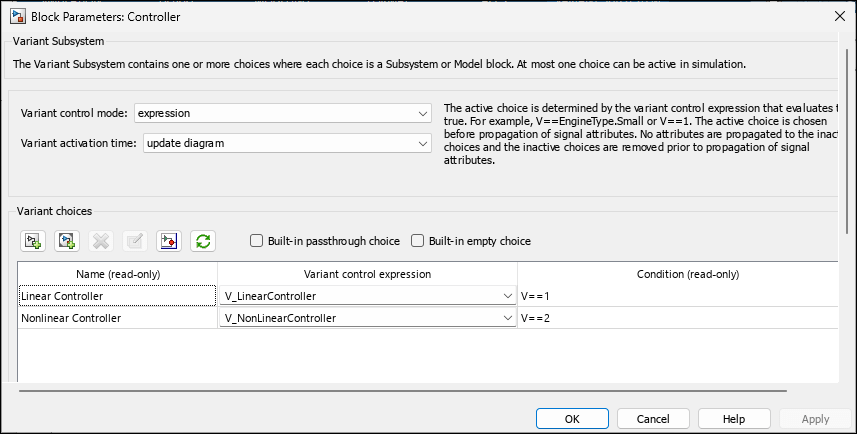Set the value of variant control variable `V` as `1` and simulate the model.

```V = 1; sim('slexVariantSubsystems');```

During simulation, the `Linear Controller` block becomes active. Double-click the `Controller` block to see the active choice. Using this approach, you can develop complex variant condition expressions that are reusable.

### Structures to Group Related Variant Control Variables of Variant Blocks

Use MATLAB® structure elements to group related variant control variables. You can control the characteristics of structures in the generated code and the placement of structures in memory. For more information, see `struct`.

Note that you can define a variant control variable of type structure only in the base workspace or in a data dictionary. Defining the structure type of variant control variables in the mask or model workspace is not supported. For more information on storage locations for variant control variables, see Storage Locations for Variant Control Variables (Operands) in Variant Blocks.

Open the `slexVariantSubsystems` model.

`open_system('slexVariantSubsystems');`
```VSS_LINEAR_CONTROLLER = VariantExpression with properties: Condition: 'VSS_MODE==1' ```
```VSS_NONLINEAR_CONTROLLER = VariantExpression with properties: Condition: 'VSS_MODE==2' ```
```VSS_MODE = 2 ```

In the base workspace, define a structure to store related variant control variables. Add fields to the structure using dot notation.

```Controller.Linear = 1; Controller.NonLinear = 0;```

In the block parameters dialog box of the `Controller` block, specify variant controls using dot notation. Also, change the Variant activation time to `code compile`.

```set_param('slexVariantSubsystems/Controller/Linear Controller', 'VariantControl', 'Controller.Linear==1'); set_param('slexVariantSubsystems/Controller/Nonlinear Controller', 'VariantControl', 'Controller.NonLinear==1'); set_param('slexVariantSubsystems/Controller', 'VariantActivationTime', 'code compile');```

Ensure that the `Linear Controller` and `NonLinear Controller` blocks are atomic.

```set_param('slexVariantSubsystems/Controller/Linear Controller', 'TreatAsAtomicUnit', 'on'); set_param('slexVariantSubsystems/Controller/Nonlinear Controller', 'TreatAsAtomicUnit', 'on');```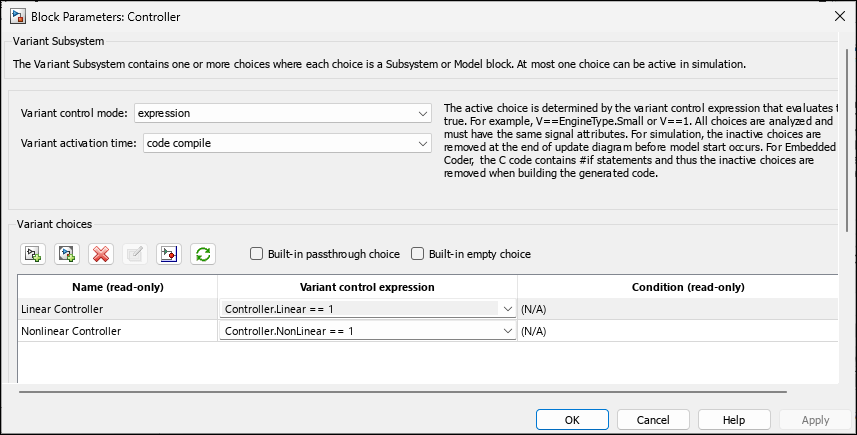Simulate the model. During simulation, the `Linear Controller` block becomes active.

`sim('slexVariantSubsystems');`

Double-click the `Controller` block to see the active choice.

Now, generate code from the model. For information on how to generate code, see Generate Code Using Embedded Coder (Embedded Coder).

The generated code contains both Linear and Nonlinear choices in the preprocessor conditionals `#if` and `#elif` because of the `code compile` activation time.

The generated header file `slexVariantSubsystems_types.h` defines a structure type that has a random name and initializes the variables `Controller_Linear` and `Controller_NonLinear`.

```% #ifndef Controller_Linear % #define Controller_Linear 1 % #endif % % #ifndef Controller_NonLinear % #define Controller_NonLinear 0 % #endif % % #ifndef DEFINED_TYPEDEF_FOR_struct_XfS20q0AY2qBc3cwjMQEtF_ % #define DEFINED_TYPEDEF_FOR_struct_XfS20q0AY2qBc3cwjMQEtF_ % % typedef struct { % real_T Linear; % real_T NonLinear; % } struct_XfS20q0AY2qBc3cwjMQEtF;```

The source file `slexVariantSubsystems.c` defines the logic for `Controller_Linear` and `Controller_NonLinear` choices.

```% void slexVariantSubsystems_step(void) % { % #if Controller_Linear == 1 % logic of Linear Controller % #elif Controller_NonLinear == 1 % logic of Nonlinear Controller % #endif % }```

For more information, see Organize Data into Structures in Generated Code (Embedded Coder).